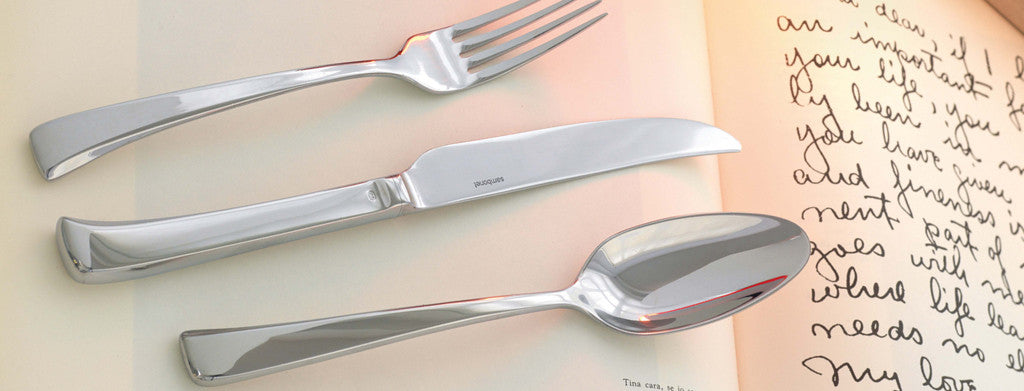Shopping Cart

### Refine

View all

#### Price# Cutlery£3.40 each (ex VAT)£3.95 each (ex VAT)£2.52 each (ex VAT)£3.29 each (ex VAT)£2.52 each (ex VAT)£3.98 each (ex VAT)£1.71 each (ex VAT)£3.40 each (ex VAT)£3.05 each (ex VAT)£3.95 each (ex VAT)£1.98 each (ex VAT)£4.89 each (ex VAT)£3.95 each (ex VAT)£3.46 each (ex VAT)£3.95 each (ex VAT)£1.43 each (ex VAT)£3.89 each (ex VAT)£4.89 each (ex VAT)£4.30 each (ex VAT)£3.05 each (ex VAT)£2.99 each (ex VAT)£4.98 each (ex VAT)£3.95 each (ex VAT)£3.46 each (ex VAT)£1.71 each (ex VAT)£3.98 each (ex VAT)£3.40 each (ex VAT)£2.63 each (ex VAT)£0.31 each (ex VAT)£0.55 each (ex VAT)£0.88 each (ex VAT)£3.95 each (ex VAT)£4.30 each (ex VAT)£2.52 each (ex VAT)£3.23 each (ex VAT)£3.26 each (ex VAT)£4.45 each (ex VAT)£0.34 each (ex VAT)£0.31 each (ex VAT)£1.04 each (ex VAT)£0.55 each (ex VAT)£0.53 each (ex VAT)£0.52 each (ex VAT)£0.97 each (ex VAT)£0.52 each (ex VAT)£1.43 each (ex VAT)£0.76 each (ex VAT)£0.59 each (ex VAT)